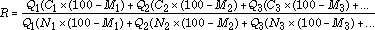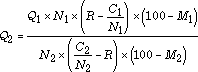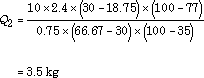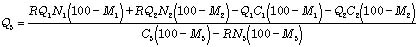## C/N Ratio

### Tom Richard and Nancy Trautmann

Once you have calculated the moisture content of your compost mixture, the other important calculation is the carbon-to-nitrogen ratio (C/N). Grass clippings and other green vegetation tend to have a higher proportion of nitrogen (and therefore a lower C/N ratio) than brown vegetation such as dried leaves or wood chips. If your compost mix is too low in nitrogen, it will not heat up. If the nitrogen proportion is too high, the compost may become too hot, killing the compost microorganisms, or it may go anaerobic, resulting in a foul-smelling mess. The usual recommended range for C/N ratios at the start of the composting process is about 30/1, but this ideal may vary depending on the bioavailability of the carbon and nitrogen. As carbon gets converted to CO2 (and assuming minimal nitrogen losses) the C/N ratio decreases during the composting process, with the ratio of finished compost typically close to 10/1.

Typical C/N ratios and nitrogen values for many kinds of compostable substances can be looked up in published tables such as Appendix A, On-Farm Composting Handbook. Some additional nitrogen and ash data is in the table of Lignin and Other Constituents of Selected Organic Materials. (A No-Frames version of the Table of Lignin is also available.) To calculate the carbon content given C/N and percent nitrogen, solve:

%C = %N x C/N

You may be able to measure the carbon and nitrogen content of your own materials and then calculate the ratio directly. Soil nutrient analysis laboratories or environmental testing laboratories can do the nitrogen test, and maybe carbon as well . Your local Cooperative Extension office can give you the names of soils laboratories in your area. The Cornell Nutrient Analysis Lab has information about their procedures for total carbon, organic carbon, and total nitrogen analysis. You can also estimate the carbon content from ash or volatile solids data if either is available. Once you have the C/N ratios for the materials you plan to compost, you can use the following formula to figure out the ratio for the mixture as a whole:in which: R = C/N ratio of compost mixture Qn = mass of material n ("as is", or "wet weight") Cn = carbon (%) of material n Nn = nitrogen (%) of material n Mn = moisture content (%) of material n

This equation can also be solved exactly for a mixture of two materials, knowing their carbon, nitrogen, and moisture contents, the C/N ratio goal, and specifying the mass of one ingredient. By simplifying and rearranging the general equation, the mass of the second material required would be:Returning to the previous example of grass and leaves, lets assume the nitrogen content of the grass is 2.4% while that of the leaves is 0.75%, and the carbon contents are 45% and 50% respectively. Simple division shows us that the C/N ratio of the grass is 18.75 and the C/N content of the leaves is 66.67% For the same 10 kg of grass we had before, if our goal is a C/N ratio of 30:1, the solution is:Note that we need only 3.5 kg leaves to balance the C/N ratio, compared with 6.8 kg leaves needed to achieve the 60% moisture goal according to our previous moisture calculation. If the leaves were wetter or had a higher C/N ratio, the difference would be even greater. So how many leaves should you add?

If we solve the general form of the C/N equation for the 10 kg of grass and the 6.8 kg of leaves (determined from the moisture calculation), and use the same values for percent moisture, C, and N, the resulting C/N ratio is a little less than 37:1. In contrast, if we solve the general form of the moisture equation for 10 kg of grass and only 3.5 kg of leaves, we get a moisture content over 66%. (To gain familiarity with using the equations, you can check these results on your own).

In this example, as is often the case, moisture is the more critical variable. This is especially true toward the wet end of the optimum (>60%), where anaerobic conditions are likely to result. So it is usually best to err on the side of a high C/N ratio, which may slow down the compost a bit but is more likely to be trouble free. If, on the other hand, your mixture is dry, then you should optimize the C/N ratio and add water as required.

As with moisture calculations, mixtures of 3 or more materials can be solved for the mass of the third material if the first two are specified (one equation & one unknown). Given the carbon, nitrogen and moisture contents of each ingredient, the masses of the first two, and the C/N ratio goal, the solution for the mass of the third material is:If we also want to consider moisture content, we can solve both equations simultaneously (moisture and C/N) for any two unknowns.